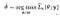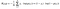# Logistic loss via Bernoulli Distribution

## Ever wondered if logistic loss can be reached via Bernoulli Distribution ?

Before we start first lets get familiar with few terminologies.

# Maximum likelihood estimation

Maximum likelihood estimation (MLE) is a method of estimating the parameters of a probability distribution by maximizing a likelihood function.

OR

In simple words it is a way of getting parameters which can maximize our model.Here y is the output and theta being parameter of model

If function is differentiable, MLE can be achieved by differentiating the model on local maxima.

# Bernoulli Distribution

What is Bernoulli Distribution ?

Bernoulli Distribution is the discrete probability distribution of a random variable which takes the value 1 with probability p and the value 0 with probability q=1-p and also a special case of binomial distribution.

OR

In simple words it gives you probability of class 1 (Positive) or 0 (Negative) based on the value of k.

# Now tell me how to reach ????

Well most of the readers would already be knowing that cross entropy and logistic loss has same mathematical formula and hence it can be said that logistic loss is achieved using binary cross entropy, so lets go in details of how to reach there via Bernoulli distribution.

Logistic loss’s mathematical form :eq0 : Binary cross entropy / Log loss / Logistic loss

Lets go step by step:-

• First we have to select a model M which can be used for improving probabilities for each class.
• As we have seen Bernoulli distribution can get us probability of class 1 or 0 so we can use this model for estimating probability for our binary classes.
• Once we have chosen our model M we can apply Maximum Likelihood Estimation on it in order to improve the probabilities by updating parameters.
• Now in order to determine the value of maximum value for M we have to differentiate and get the value of k on local maxima.
• To make differentiation simpler we can apply log on both sides of eq1.
• Now after differentiating eq2 we can get the optimal value of k.
• If we compare eq0 with eq2 both are almost same except the negative sign and the number of iteration (N) term.
• Hence if we put a negative sign (-) on eq2 along with summation over N iterations it will become something like Minimum Likelihood Estimation and finally it can be used as loss function.